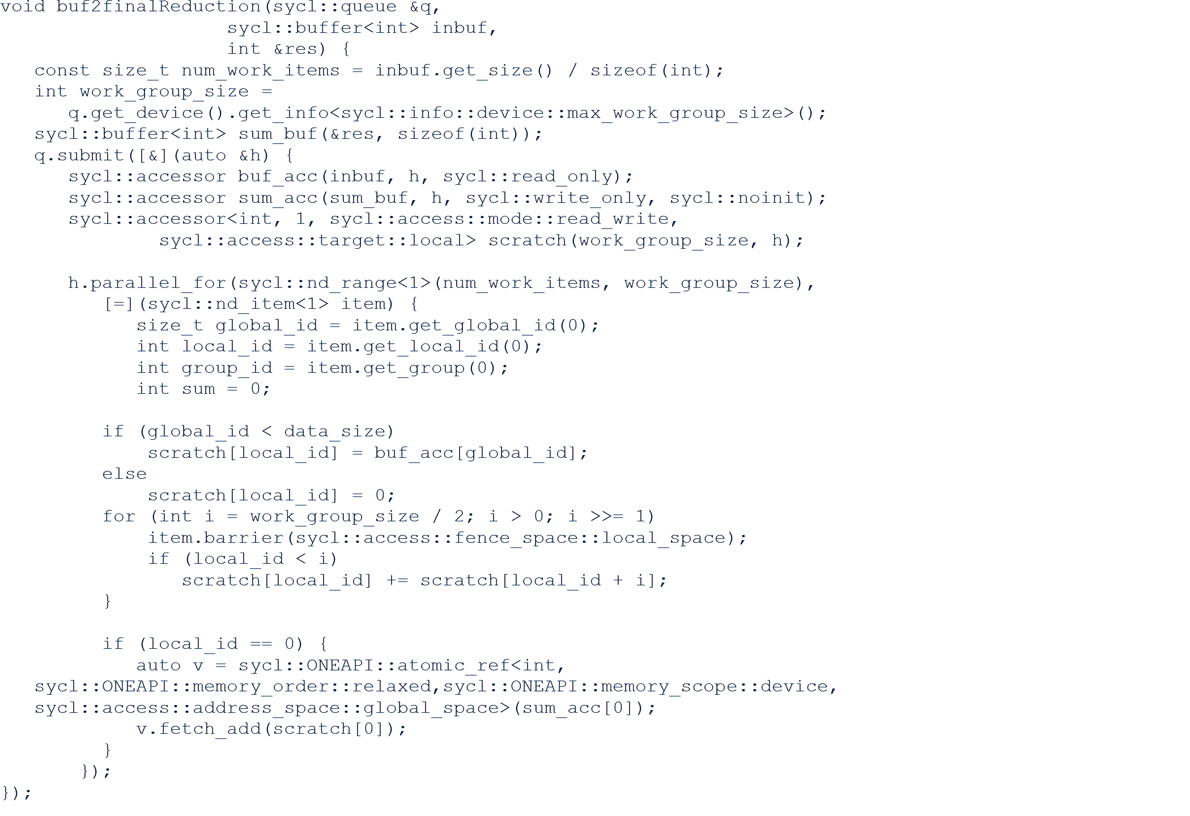# Reduction Operations in Data Parallel C++

ID 676385
Updated 4/20/2021
Version Latest
PublicBy

Implementing and Tuning the Common Reduction Parallel Pattern

Ramesh Peri, senior principal engineer, Intel Corporation
@IntelDevTools

Get the Latest on All Things CODE

## An Introduction to the Reduction Operation

Reduction is a common operation in parallel programming that reduces the elements of an array into a single result. Let’s say you want to add the elements of a large array into a single sum (Figure 1). Doing this operation in parallel requires the computation of partial sums that are sequentially combined, or reduced, to produce the final result. Reduction operators (for example, summation, minimum, maximum, minimum location, and maximum location) are associative and are often commutative. There are many ways to implement a reduction, and its performance depends on the underlying processor architecture. This article explores several ways to express reductions in Data Parallel C++ (DPC++) and discusses the performance implications of each.

﻿﻿

Figure 1. Pictorial representation of a summation reduction

## Reduction in DPC++ Using Global Atomics

In the following implementation, each work item in the DPC++ kernel is responsible for an element of the input array and atomically updates a global variable:Depending on the number of threads created by the compiler (which in turn depends on the default workgroup and subgroup sizes chosen by the compiler for the target device), the contention for accessing the single global variable, sum_buf, will be quite high. In general, the performance of such a solution will not be very good.

## Reducing Contention on Global Atomics

One way to reduce the contention on the global variable update is to decrease the number of threads accessing this variable. This can be achieved by making each work item process multiple elements of the array, perform a local reduction on a chunk of elements, and then perform the global atomic update (Figure 2).

﻿﻿

Figure 2. Each work item computes a partial sum

This implementation is shown in the following code:This implementation is not very efficient because each work item is accessing contiguous locations in memory, which causes the compiler to generate inefficient code. The DPC++ compiler treats each work item like a vector lane, so work items accessing contiguous locations of memory will be inefficient.

## Efficient Access of Memory by Work Item

The DPC++ compiler maps each work item to a vector lane of the underlying processor, which allows the compiler to generate efficient code when the work items access memory locations with a stride greater than the vector length of the processor. This access pattern is shown in Figure 3, where each of the labels (wi-1, wi-2, and so on) represent each of the n work items.

﻿﻿

Figure 3. Each work item computes a partial sum from noncontiguous memory locations

The kernel where each work item operates on multiple, interleaved elements of the input vector is shown in the following code:The choice of number of work items is important. It is selected to be the product of the number of processing elements and the preferred vector width of the processing element. This number may be sufficient on some platforms, but perhaps not for others where the number of threads supported is much larger than the number of processing elements. Consequently, this number must be chosen carefully based on the target platform.

## Tree Reduction

A tree reduction is a popular technique in which each of the work items in a kernel apply the reduction operator to adjacent elements, producing intermediate results with multiple levels. This can be applied within a workgroup, as shown in Figure 4, because thread scheduling and synchronization are highly efficient, with hardware support within a workgroup.

﻿﻿

Figure 4. Tree reduction

The size of a workgroup is fairly small (about 256 or 512) and is dependent on the hardware device, while the number of elements to be reduced is an order of magnitude larger than the workgroup size. This means that the reduction operation performed by each workgroup produces an intermediate result that needs to be further reduced. This can be handled in two ways:

1. By each workgroup calling a global atomic add with its intermediate result, as shown in the following code:2. By calling the same reduction kernel once again on the list of intermediate values produced by the workgroups to produce another set of intermediate values. At some point, once the number of intermediate results is small enough, one can simply use the global atomic updates instead of calling the kernel to get the final result.
3. The kernel implementing this technique is shown below. Here, the output of the kernel is another set of values that are stored by each workgroup in the output buffer.The previous two kernels can be called as shown below to get the final result. Here, X is the size of the intermediate result where the final atomics-based reduction is called, and this value is chosen based on the efficiency of the global atomics-based reduction.## DPC++ Built-In Reduction Operator

DPC++ provides a summation reduction operator, which is used in the kernel below. In this case, the compiler chooses an implementation that is the most efficient for the underlying platform. It is usually recommended to use the built-in reduction operator.## Final Thoughts

Reduction is a common parallel programming pattern used in many applications. This article explored some ways of implementing this operation in DPC++. The performance of these implementations can be quite different depending on the compiler and the target platform. The next article explores the performance of these kernels on CPUs and GPUs.

## You May Also Like

Get the Software

### Intel® oneAPI Base Toolkit

Get started with this core set of tools and libraries for developing high-performance, data-centric applications across diverse architectures. See All Tools